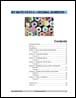## My Math Path: 4, 5 and 6 - Decimal numbers, Common fractions and Powers & roots (2011)This is an e-learning textbook for adults who want to upgrade their basic math skills, and for teachers or tutors looking for math resources they can use with students.

The first section covers decimals in general, with specific reference to place value; mathematical operations with decimals; and order of operations.

The second section of the textbook covers fractions and includes material on operations with fractions; equivalent fractions; converting between fractions and decimals; and estimation.

The third section is subdivided into modules on ratios and proportions; percent; powers and roots; and statistics.

Each section or module begins with a statement of learning outcomes; a glossary of terms used; numerous math exercises; and a practice test and solutions.

Authors:
Subjects:
Funders: